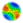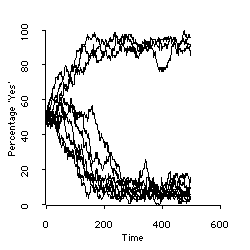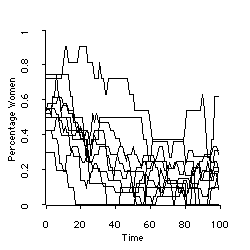Nigel Gilbert (1999)

Multi-level simulation in Lisp-Stat

Journal of Artificial Societies and Social Simulation vol. 2, no. 1, <https://www.jasss.org/2/1/3.html>

To cite articles published in the Journal of Artificial Societies and Social Simulation, please reference the above information and include paragraph numbers if necessary###Abstract

A package of Lisp functions is described which implements a simple multi-level simulation toolkit, MLS. Its design owes a great deal to MIMOSE. MLS runs within Lisp-Stat. It offers a set of functions, macros and objects designed to make the specification of multi-level models straightforward and easy to understand. Lisp-Stat provides a Lisp environment, statistical functions and easy to use graphics, such as histograms, scatterplots and spin-plots, to make the results of multi-level simulations easy to visualise.

Keywords:
Multi-level simulation, Social simulation, Lisp, Computer modelling, Social simulation tookit

###Introduction

1.1
Multi-level simulation consists of simulating interacting populations where the population attributes depend on aggregated individual attributes and individual attributes depend on the population attributes (see Troitzsch 1996 for a tutorial introduction). This package implements a simple multi-level simulation toolkit, MLS. Its design is based on the functionality of MIMOSE (Moehring, 1996). MLS runs within Lisp-Stat (Tierney, 1990). It offers a set of functions, macros and objects designed to make the specification of multi-level models straightforward and easy to understand. Lisp-Stat provides a Lisp environment, statistical functions and easy to use graphics, such as histograms, scatterplots and spin-plots, to make the results of multi-level simulations easy to visualise. Lisp-Stat is available for Macintosh, PC and Unix computers and works almost identically on each. It can be downloaded from several sites on the Internet.

1.2
The MLS package consists of three files: the toolkit itself, mls.lsp, and two examples: a barebones interacting population example, interpop.lsp, and a simulation of a state education system, teachers.lsp.

1.3
After starting Lisp-Stat, compile and load the toolkit by evaluating the expression:

`(compile-file "mls" :load t)`

###Creating an MLS model

2.1
There are four steps to create a MLS model:

1. Specify the objects in the model. There will be a hierarchy of objects, one or more at each level. For example, one might have as objects: the state, school, teacher, student in a four level model of an education system.
2. Specify the processes which the objects undergo during the passage of simulated time.
3. Specify the initial conditions
4. Specify a function to run the model and graphs etc. to display the output.

The model can then be run.

Each of the steps will now be described in more detail.

#### 1. Specify the objects in the model

For each object, you need to specify its attributes (if any). For example, a teacher might have the attributes age, sex and status. The teacher object could be defined by typing in the Lisp-Stat listener window:

```(defobject teacher (age sex status)) ```

Alternatively, this could be typed into a file and the file loaded into Lisp-Stat. Another example:

```(defobject student (sex maths-mark)) ```

The general form for defining an object is:

```(defobject name (list-of-attributes) (list-of-temporary-variables)) ```

Both the list-of-attributes and the list-of-temporary-variables may be omitted.

A history is automatically kept of the values of all attributes. Thus, for instance, you can access the status of a teacher two time steps ago, or display a graph showing the changing status of a teacher since the beginning of the simulation. Temporary variables differ from attributes in that no history of their values is kept.

#### 2. Specify the processes which objects undergo

Each object in the simulation can carry out some computation every time step. This computation should be specified in a method called :act. So, for example, if we want each teacher to become one year older at each time step, we would define an :act method for teachers thus:

```(defmeth teacher :act () (set-attribute age (+ (prev-attribute age) 1))) ```

This assumes that the object teacher has already been defined and that it has been given the attribute age.

The example above uses two MLS functions. Set-attribute sets the value of an attribute. Prev-attribute recovers the value of the attribute at a previous time step.

The general syntax of these functions is:

`(set-attribute name-of-attribute new-value)`

`(prev-attribute name-of-attribute &optional (time-steps 1))`

&optional (time-steps 1) means that the second argument, time-steps, can be omitted and if it is, it takes the value 1 by default.

Another example of the :act method is:

```(defmeth student :act () (set-attribute maths-mark (normal 60 10)))```

The function (normal mean st_dev) returns a number selected from a normal distribution with the given mean and standard deviation.

The function

`(attribute name-of-attribute) `

returns the current value of an attribute.

The functions mentioned so far access the attributes of the object whose behaviour is being defined (the teacher or the student, in the examples). There are also functions which access the attributes of other objects:

`(its-attribute object name-of-attribute) `

`(its-prev-attribute object name-of-attribute &optional (time-steps 1))`

#### 3. Specify the initial conditions

The state of each object at the start of the simulation needs to be specified. In particular, some objects will have other objects at a lower level associated with them. For example, a teacher might be initialised to have a class of students under its control.

Initialisation is specified by defining an :initialise method for the object. Here is an example of an initialise method for a teacher which creates a class of 20 students (the method assumes that the object student has previously been defined, using defobject):

```(defmeth teacher :initialise () (create-lowers student 20))```

The effect of this is to create 20 student objects as the teacher's "lower objects", that is, on the next lower level of analysis. As part of the creation of each student, the students' own :initialise method will be evaluated, so we had better define this too:

```(defmeth student :initialise () (set-attribute sex (randomly-choose 'male 'female)))```

This method randomly assigns a sex, male or female, to the student. The method does not create any lower objects: students are at the bottom of the hierarchy of levels in this example.

The :initialise method may set any of the attributes of the initialised object. If attributes are not set explicitly, they are initialised to nil.

#### 4. Specify a function to run the model and graphs etc. to display the output

Finally, a function needs to be written to set the simulation in motion. This function needs to do three things:

First, create the top level object. For example, in a simulation with school, teacher, and student objects, the function will need to create a school object. This will automatically be initialised as it is created, and its initialise method should have been written so as to create and initialise the school's teachers. These in turn will create and initialise the students, so creating a school has the effect of creating the teachers and students within it.

To create a new object, evaluate the function create on it, for example:

`(create school)`

It might be useful to control how many classes there are in the school and how big each class in the school is. This can be done by supplying an extra argument to the create function, and defining the :initialise methods appropriately. For instance:

```(defmeth school :initialise (classes class-size) (create-lowers teachers classes class-size))```

``` (defmeth teacher :initialise (class-size) (create-lowers students class-size))```

Now to create a school with 5 classes, each of 25 students, evaluate:

``` (create school 5 25)```

Second, the simulation needs to be driven forwards through time. This is done by sending the message :step to the top level object. For example, the following fragment runs the school simulatuon through twenty steps:

```(dotimes (s 20) (send school :step))```

Third, the results of the simulation need to be output. Often, one wants to observe the behaviour of all the objects in the simulation. To find the objects, you have to start with the top-level object and obtain from it the identities of the objects at the next level down. For example,

`(send school: lowers)`

returns a list of the teacher objects in the school. Each of the teacher objects can then be sent the same message to obtain lists of the students in their classes.

Once you have a handle on an object, such as a particular student, you can obtain a record of the changing values of any of its attributes. The function its-history is used for this.

`(its-history object name-of-attribute)`

returns a list of the successive values of the given attribute of the object over the course of the simulation.

For example, to find the maths mark at each time step of the student whose object is in the variable a-student, use

```(its-history a-student maths-mark) ```

The two example files, interpop.lsp and teachers.lsp, include functions showing how the output from this function can be plotted using the Lisp-Stat plot functions.

2.2
To demonstrate the package in use, two examples have been coded from the descriptions provided in Troitzsch's (1996) tutorial paper. The first (interpop.lsp) shows opinion formation in a homogeneous population. One hundred people have to make a decision, either 'yes' or 'no'. Initially, opinion is equally and randomly disttributed through the population. Individuals then change their opinion according to a formula which includes a coupling coefficient that indicates how strongly the individual's opinion is tied to that of the prevailing majority. Figure 1 shows the results for 10 runs, using a coupling coefficient (kappa) of 1.5. The populations all tend to around either 10 or 90 per cent 'yes' votes, with a similar proportion going to each end point.Figure 1: Bimodal trends in opinion formation in a homogeneous population

2.3
The second example (teachers.lsp) is taken from Troitzsch's (1996) work on gender desegregation in German gymnasia. The simulation is based on the assumptions that teachers leaving their jobs are replaced by men and women with equal overall probability; men stay in their jobs twice as long as women; and new women teachers are assigned to an individual school with a probability which depends on the percentage of women among its teachers. Figure 2 shows the resulting distribution of women teachers to schools for a run involving ten schools, each having an average of 20 teachers.Figure 2: Simulated distribution of percentages of women among teachers at 10 schools

###Tracing in MLS

3.1
To see what is happening as the simulation runs, you can specify that a message is printed in the Listener window every time the value of a particular attribute changes.

3.2
For example, to trace the changing maths-marks of students, evaluate

`(mls-trace maths-mark)`

The output will look like

`Attribute MATHS-MARK of #<STUDENT-0> set to 57.829241873061605 `

Any number of attributes may be given in one call of mls-trace. To stop tracing an attribute, evaluate mls-untrace with the name of the attribute, or (mls-untrace) to stop all tracing.

###Additional functions in MLS

4.1
In addition to the functions and methods mentioned above, the following are provided:

:from-lowers
This method returns a list of the values of a given attribute from all the objects at the next lower level. For example,

`(send a-teacher :from-lowers 'maths-mark)`

would return a list of all the math marks of the students in a-teacher's class.

:num-lowers
This method returns the number of objects at the next lower level. For example,

`(send a-teacher :num-lowers)`

returns the number of students in a-teacher's class.

`(upper-attribute name-of-attribute)`

This function returns the value of the given attribute of the object next up in the hierarchy. For example, when evaluated within the body of a method defined for the student object,

`(upper-attribute 'sex)`

would return the sex of the student's teacher.

`(prev-upper-attribute name-of-attribute &optional (time-steps 1))`
This function returns the value at the previous time step of the given attribute of the object next up in the hierarchy.

###Random functions

5.1
In addition to the large number of functions provided by Lisp-Stat for generating random numbers from distributions, MLS offers the following:
`(uniform lo-bound hi-bound)`
returns a random number between low and high bounds, drawn from a uniform distribution.

`(normal mean standard-deviation)`
returns a number drawn from a normal distribution with the given mean and standard deviation.

`(chance odds)`
returns true with a probability of 1 in the given odds. Odds must be an integer greater than 1. For example, (chance 3) will return true once every three times it is evaluated, on average.

`(randomly-choose options...)`
returns one of the options at random, each with equal probability. For example,

`(randomly-choose lust gluttony envy pride)`

will return one sin chosen randomly from the list.

`(prob probability)`
returns true with the given probability (a number less than 1). For example, `(prob 0.5)` returns true half the time.###References

MOEHRING, M. (1996). Social Science Multilevel Simulation with MIMOSE, in K.G. Troitzsch, U. Mueller, G.N. Gilbert and J.E. Doran, Social Science Microsimulation Berlin: Springer

TIERNEY, L (1990) Lisp-Stat: an object-orientated environment for statistical computing and dynamic graphics, London: Wiley

TROITZSCH, Klaus G. (1996). Multilevel simulation, in K.G. Troitzsch, U. Mueller, G.N. Gilbert and J.E. Doran, Social Science Microsimulation Berlin: Springer